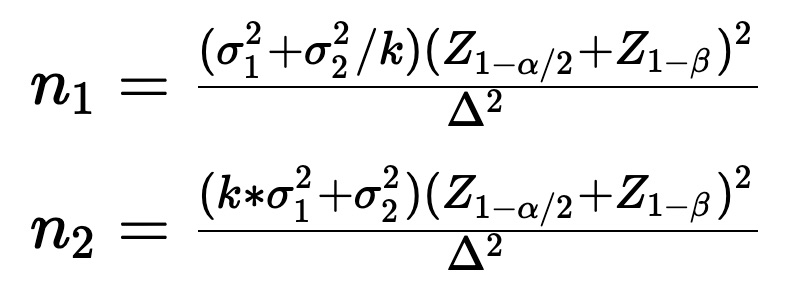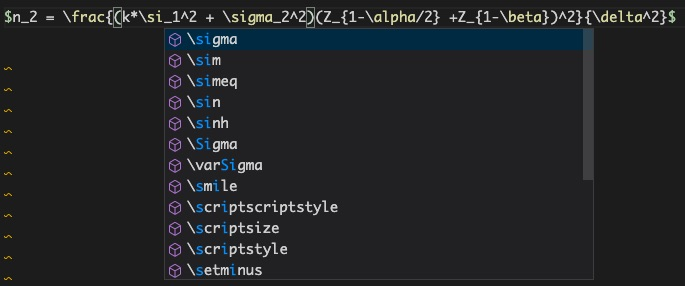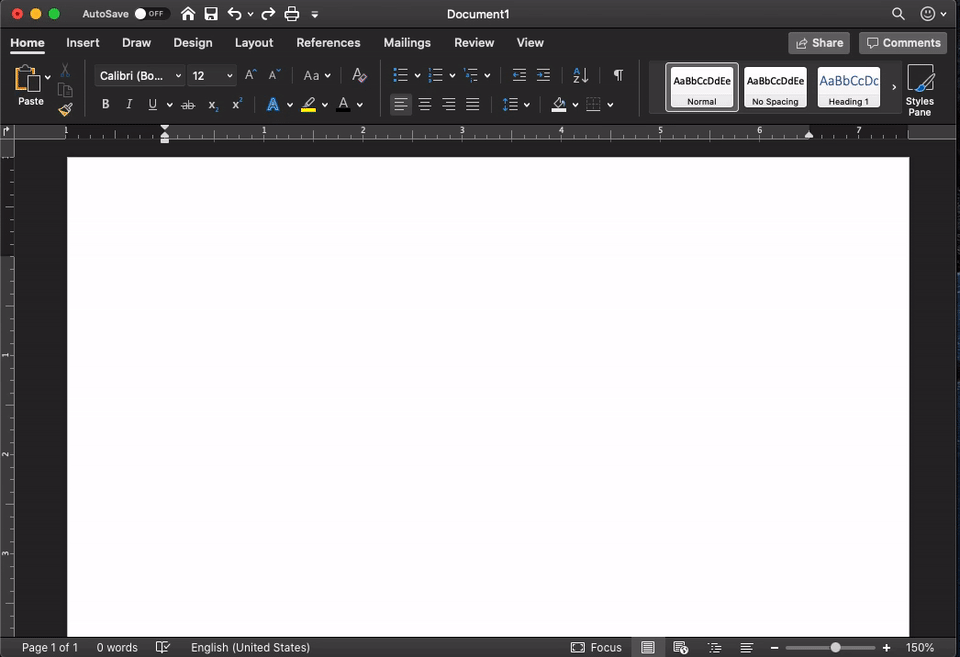# Embedding LaTeX Equations in MS Word

I have got used to Markdown for most of my notes and sometimes even for delivering presentations at work. I unwillingly returned to Word to help out my wife on one of her tasks related to her studies. I had to insert some statistics related formula in Word. Its been ages I played with that section of Word so it took some time to figure out. After playing around with the equations and symbols for few minutes, I got so frustrated with the convoluted workflow of inserting various math symbols and that too with different subscripts and superscripts. I basically FU to word and returned to Markdown to insert the equation using MathJax. The equations goes some like this:With Markdown, I can pretty much type this equation in natural language in a flow. Intellisense in VS Code works great here as well as it provides auto suggestion and completion as I type. The text for the first equation in the above image maps to:

$n_1 = \frac{(\sigma_1^2 + \sigma_2^2/k)(Z_{1-\alpha/2} +Z_{1-\beta})^2}{\delta^2}$


The initial ramp with LaTeX equation may be little steep, but it pays off handsomely in the long run. We can type an equation very similar to how we spell it out. Here is a snapshot from my VS Code.Later I found out that Word also had a short cut to convert LaTeX equations into Word equations. That came in so handy to move the equations from my markdown notes directly to Word without going through that difficult workflow. With minor edits (such as replacing {} with ()), it was so easy to convert these equations into a readable one.Shortly later, as I explored more, I found out that Word also supports a similar type to convert. Explained in this link. It worked good as typed in the equation box. I still liked the flexibility to the equations into professional format due its multipurpose.

Tags:

Categories:

Updated: